# SALR potential

The SALR ( short-range attractive - long-range repulsive) potential. This potential has a variety of functional forms.

## HCDY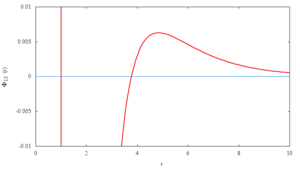Plot of a region of the HCDY potential for the parameters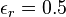$\epsilon_r=0.5$,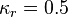$\kappa_r=0.5$,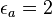$\epsilon_a=2$,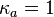$\kappa_a=1$, as used in the work of Sweatman et. al. 

The SALR potential is often expressed as a hard core of diameter$\sigma$ , along with combination of two Yukawa potentials: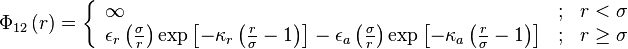$\Phi_{12}\left( r \right) = \left\{ \begin{array}{lll} \infty & ; & r < \sigma \\ \epsilon_r \left(\frac{ \sigma }{r}\right) \exp \left[- \kappa_r \left( \frac{r}{\sigma} - 1 \right) \right] - \epsilon_a \left( \frac{ \sigma }{r}\right) \exp \left[- \kappa_a \left( \frac{r}{\sigma} - 1 \right) \right] & ; & r \ge \sigma \end{array} \right.$

where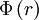$\Phi\left( r \right)$ is the intermolecular pair potential,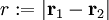$r := |\mathbf{r}_1 - \mathbf{r}_2|$ is the distance between site 1 and site 2. The$\epsilon$ control is the energy of the repulsive and attractive parts, whilst the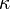$\kappa$ controls the interaction range.

This potential also goes by the names of the hard-sphere plus two Yukawa (H2Y) or hard-core double-Yukawa (HCDY) potential  .

## LJ+Y

Another SALR model consists of a generalised Lennard-Jones model in conjunction with a long-range repulsive Yukawa term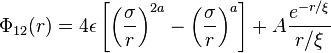$\Phi_{12}(r) = 4 \epsilon \left[ \left(\frac{\sigma}{r} \right)^{2a}- \left( \frac{\sigma}{r}\right)^a \right] + A \frac{e^{-r/\xi}}{r/\xi}$